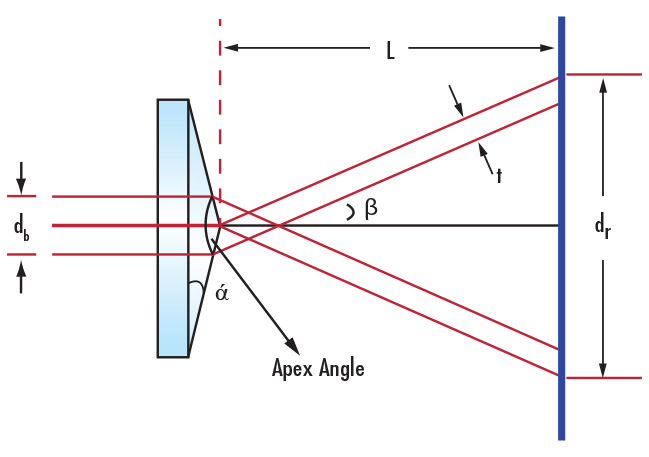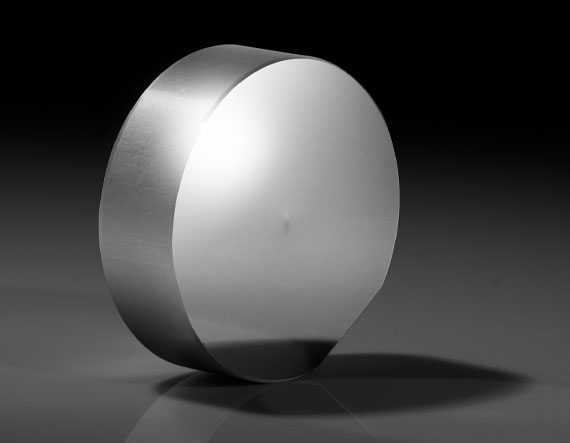# Axicon Calculator

View All Technical Tools

db (mm):
L (mm):
n:
Axicon Angle (°):

dr (mm) (Diameter of the Ring): --

t (mm) (Line Thickness of the Ring): --

β (°) (Half Fan Angle): --$$d_r = 2L \cdot \tan{\left[ \left( n - 1 \right) \alpha \right]}$$
 $$\beta = \sin^{-1}{\left( n \, \sin{\alpha} \right)} - \alpha$$
 $$t = \frac{1}{2} d_b$$
 dr: Outer diameter of the ring that the beam forms db: Diameter of the beam that enters the lens t: Thickness of the line that the beam forms β: Half fan angle that beam forms
 L: Length from Axicon to image formed n: Refractive index of the Axicon α: Axicon angle
Units are in mm

The calculator functions by taking the known variables and using the equations to determine the unknowns of the Axicon.

The figure above demonstrates the original beam entering the Axicon. It then shows how the beam exits the Axicon. The figure has arrows leading the user to the different variables used in the Axicon's formulas.

The calculator uses three equations to determine the diameter of the ring, the line thickness of the ring, and the half fan angle.

## Description

Axicons are conical prisms that are defined by the alpha and apex angle. As the distance from the Axicon to the image increases, the diameter of the ring increases, while the line thickness remains constant.

Given the input is a collimated beam, you can calculate the outer ring diameter and the line thickness an Axicon will produce. The half fan angle calculation will be an approximation.

## Related Resources and Products